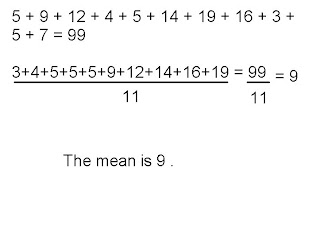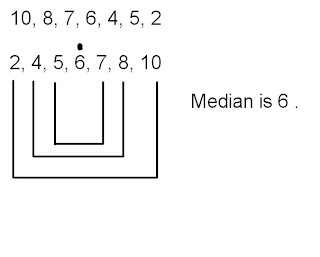### Ronnil's Measures of Central Tendancy

Monday, September 29, 2008
Mean
Arrange the data in ascending numberical order.
Add up all the data and divide the sum by the amount of data.Median
Arrange the data in ascending numberical order.
Cross out the same amount of numbers from outside in.
If there are two medians in the middle then add them both together and divide by 2.Mode
Arrange the data ascending numberical order.
The most common number in the data. ( 5, 5, 6, 7, 8, 8, 8 )Range
Arrange the data ascending numberical order.
Subract the smallest number to the biggestHeres a video of MMM.